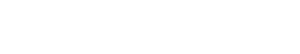# Normal Distribution

Normal distribution is a probability distribution that is symmetric around the mean, showing that data near the mean are more frequent in occurrence than data far from the mean. In graph form, normal distribution will appear as a bell curve.

## Normal Distribution vs. Gaussian Distribution vs. Bell Curve

Normal distribution is often confused with other similar terms, such as Gaussian distribution and bell curve. However, there are important differences between these terms. Gaussian distribution is a type of continuous probability distribution that is often used in the field of statistics. Bell curve is a graphical representation of data that shows how frequently data points fall within certain ranges.

While normal distribution, Gaussian distribution, and bell curve are all related, it is important to understand the differences between them to correctly apply these concepts in data analysis.

## Normal Distribution Properties

There are several properties of normal distribution that are important to understand. First, normal distribution is symmetric around the mean. This means that data points near the mean are more frequent in occurrence than data points far from the mean. Second, normal distribution is continuous, which means that there is an infinite number of possible values that a data point can take. Finally, normal distribution is defined by its mean and standard deviation. The mean is the average of all the data points, and the standard deviation is a measure of how spread out the data points are from the mean.

## Normal Distribution Empirical Rule

One of the most important properties of normal distribution is the empirical rule. The empirical rule states that for data that is normally distributed, 68% of the data will fall within one standard deviation of the mean, 95% of the data will fall within two standard deviations of the mean, and 99.7% of the data will fall within three standard deviations of the mean.

This rule is important because it allows us to make predictions about how data will behave. For example, if we know that a data set is normally distributed, we can use the empirical rule to predict how many data points will fall within certain ranges.

## Applications of Normal Distribution

Normal distribution is used in many different fields, including finance, medicine, and text analytics. In finance, normal distribution is often used to model stock prices. In medicine, normal distribution is used to model the distribution of various diseases. In text analytics, normal distribution is used to evaluate the importance of a word in a document.Unlock the power of actionable insights with AI-based natural language processing.